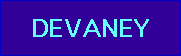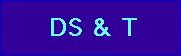Mathematical Glossary

# The Mandelbrot Set Explorer## Mathematical Glossary.

Cycle (of period n). An orbit that repeats itself every n iterations. For example, 0 lies on a cycle of period 2 for x2 - 1 since its orbit is

0 --> -1 --> 0 --> -1 ...

An n-cycle would assume the form

x0 -->x1 -->x2 --> ... --> xn-1 -->x0 --> x1 ...

Fate of the orbit of 0. The orbit of 0 is the collection of all points (complex numbers) under iteration of x2 + c starting at the seed 0. The fate of the orbit is its eventual behavior. For example, the orbit may tend to a cycle of some period---its fate would then be that cycle. Or it ---may tend to a fixed point. Its fate would then be that fixed --> --point. Or its fate could be that it tends to infinity.

Filled Julia Set. The set of all seeds whose orbits do not escape to infinity under iteration of x2 + c.

x1 = x02 + c
x2 = x12 + c
x3 = x22 + c
x4 = x32 + c

where the seed x0 is a real or complex number. We also write this as

x0 -->x1 -->x2 --> ... --> xn-1 -->xn --> ...

Mandelbrot set. The set of complex c-values for which the orbit of 0 does not escape under iteration of x2 + c. Equivalently, the Mandelbrot set is the set of c-values for which the filled Julia set of x2 + c is a connected set.

Orbit of 0 under x2 + c. The orbit of 0 is the list of complex numbers given by

0 --> c --> c2 + c --> (c2 + c)2 + c -->...

That is, successive complex numbers in this list are derived by squaring the previous entry and adding c.

Period of the Bulb. Each bulb in the Mandelbrot set consists of a set of c-values for which the corresponding quadratic function has an attracting cycle of a given period. That period is the period of the bulb.

Primary Bulb. A primary bulb is one of the infinitely many disk-like bulbs that are attached to the main cardioid in the Mandelbrot set. The bulb does not included the sub-bulbs attached to it, nor does it include the intricate antenna-like structures that protrude from the collections of secondary bulbs.Devaney home pageDynamical Systems and Technology Project Home Page